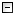Home Control Panel Login Register Search Submit Logout About me Contact meMy ResearchWeb This Site
 Home > My Research > Dynamic Models
 Category: Dynamic Models Dynamic factor models. Supplement materials for the paper \"Comparisons of Four Methods for Estimating a Dynamic Factor Model\" and  \"Bayesian Estimation of Categorical Dynamic Factor Models\". Questions can be asked at http://www.statisticalexperts.com/forum/forumdisplay.php?fid=36

Contents
 The R function to simulate data for the direct autoregressive factor score model. This is a supplement for the paper "Comparisons of Four Methods for Estimating a Dynamic Factor Model".
 Methods to be compared: 1. Bayesian estimation 2. MLE using the Kalman filter 3. MLE using the block-Toeplitz matrix 4. Least squares using the block-Toeplitz matrix
 Abstract Dynamic factor models have been used to analyze continuous time series data. Motivated by a categorical time series data set, we extend two main kinds of dynamic factor models ?C the direct autoregressive factor score (DAFS) model and the white noise factor score (WNFS) model ?C to categorical DAFS and WNFS models in the framework of the underlying variable method. To estimate the categorical dynamic factor models, a Bayesian method via Gibbs sampling is used. The applications of the proposed models are demonstrated with an empirical data set. The validities of results from the empirical study are then evaluated by means of simulation studies. Differences between continuous and categorical dynamic factor models are evaluated and discussed.
 Total 7 ,Display 1 - 7 1
 Copyright © 2003-13 Zhiyong Zhang \'s Psychometric WebsiteMaintained by Zhiyong Zhang